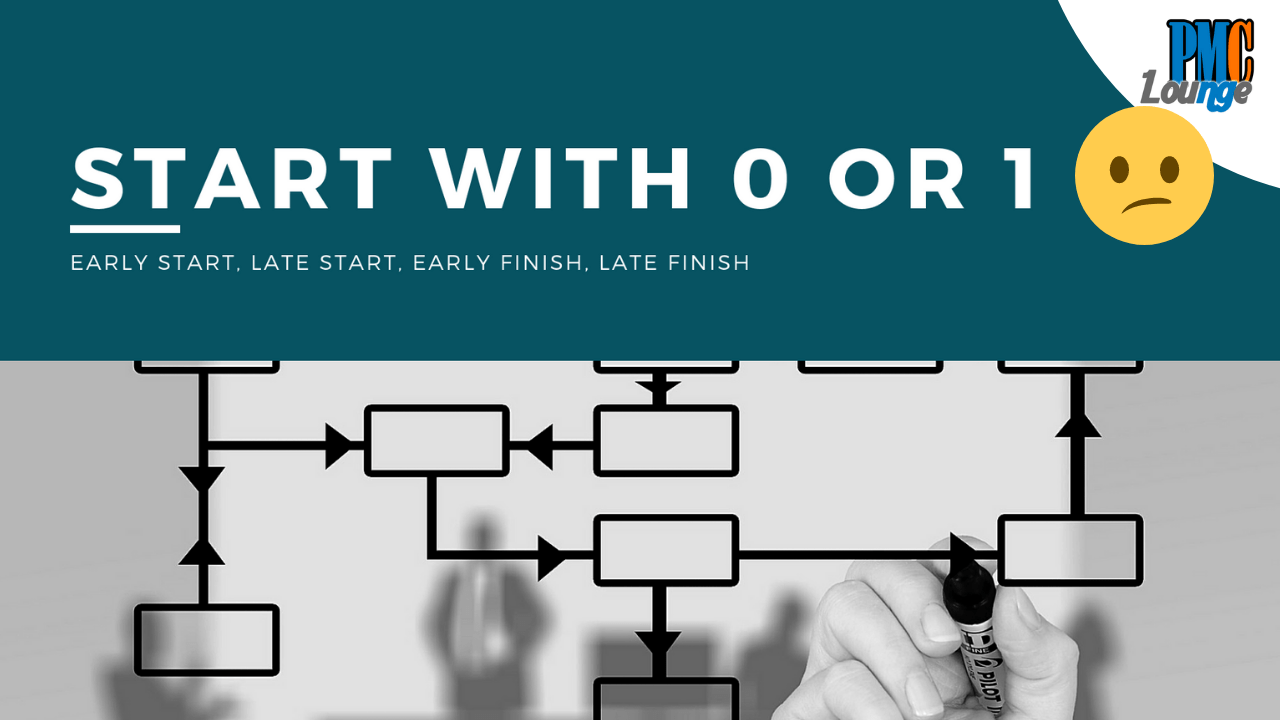Two ways to calculate the Network Diagram valuesBefore we begin with this article, it is a mandatory requirement that you know the topics below,

Or check out this playlist – https://goo.gl/S6RKCB

Now that you know how to calculate Early Start, Early Finish, Late Start and Late Finish specifically from topic number 4, it is important to know that there are actually two ways to calculate these values,

First approach – You calculate the network diagram starting on day 0. This is what we have discussed previously

Second approach – You calculate the network diagram starting on day 1. This is what we have discussed in this video. The formulas used are below,

Early Start = EF of predecessor activity + 1

Early Finish = ES + activity duration – 1

Late Finish = LS of successor activity – 1

Late Start = LF – activity duration + 1

Do refer to the video for step-by-step calculation of these values in the example below,

Check more articles on Schedule Management